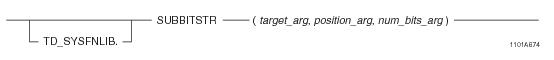# 15.00 - SUBBITSTR - Teradata Database

## Teradata Database SQL Functions, Operators, Expressions, and Predicates

Product
Release Number
15.00
Content Type
Programming Reference
Publication ID
B035-1145-015K
Language
English (United States)

## SUBBITSTR

### Purpose

Extracts a bit substring from the target_arg input expression based on the specified bit position.

### Syntaxwhere:

 Syntax element… Specifies… TD_SYSFNLIB the name of the database where the function is located. target_arg a numeric or variable byte expression. position_arg an integer expression indicating the starting position of the bit substring to be extracted. num_bits_arg an integer expression indicating the length of the bit substring to be extracted. This specifies the number of bits for the function to return.

### ANSI Compliance

This is a Teradata extension to the ANSI SQL:2011 standard.

### Description

SUBBITSTR extracts a bit substring from the target_arg string expression starting at the bit position specified by position_arg. See “Bit and Byte Numbering Model” on page 305 for the range of bit positions for each data type.

The num_bits_arg value specifies the length of the bit substring to be extracted and indicates the number of bits that the function should return. Note that since the return value of the function is a VARBYTE string, the number of bits returned will be rounded to the byte boundary greater than the number of bits requested.

The bits returned will be right-justified, and the excess bits (those exceeding the requested number of bits) will be filled with zeroes.

If position_arg is negative or out-of-range (meaning that it exceeds the size of target_arg), an error is returned.

If num_bits_arg is negative, or is greater than the number of bits remaining once the starting position_arg is taken into account, an error is returned.

If any of the input arguments is NULL, the function returns NULL.

### Argument Types and Rules

SUBBITSTR is an overloaded scalar function. It is defined with the following parameter data types for the following (target_arg, position_arg, num_bits_arg) input combinations:

 target_arg type position_arg type num_bits_arg type BYTEINT INTEGER INTEGER SMALLINT INTEGER INTEGER INTEGER INTEGER INTEGER BIGINT INTEGER INTEGER VARBYTE(n) INTEGER INTEGER

The maximum supported size (n) for VARBYTE is 8192 bytes.

All expressions passed to this function must either match these declared data types or can be converted to these types using the implicit data type conversion rules that apply to UDFs.

Note: The UDF implicit type conversion rules are more restrictive than the implicit type conversion rules normally used by Teradata Database. If any argument cannot be converted to one of the declared data types by following UDF implicit conversion rules, it must be explicitly cast. For details, see “Compatible Types” and “Parameter Types in Overloaded Functions” in SQL External Routine Programming.

If any argument cannot be converted to one of the declared data types, an error is returned indicating that no function exists that matches the DML UDF expression submitted.

### Result Type and Attributes

The result data type is a VARBYTE string. The size (number of bytes) of the VARBYTE string depends on the data type of the target_arg input argument and the number of bits requested.

For example:

 IF the data type of target_arg is... THEN the result type is... AND the result format is the default format for... BYTEINT VARBYTE(1) VARBYTE(1) SMALLINT VARBYTE(2) VARBYTE(2) INTEGER VARBYTE(4) VARBYTE(4) BIGINT VARBYTE(8) VARBYTE(8) VARBYTE(n) VARBYTE(m) where m is the smallest number of bytes to accommodate the requested number of bits. VARBYTE(m)

The maximum supported size (n) for VARBYTE is 8192 bytes.

The default title for SUBBITSTR is: SUBBITSTR(target_arg, position_arg, num_bits_arg).

For information on default data type formats, see SQL Data Types and Literals.

### Example

The following query takes the input argument 20, which has a data type of BYTEINT and a binary representation of 00010100, and requests that 3 bits be returned starting at the third bit. The 3 bits returned are 101, which are placed into a right-justified zero-filled byte. The result from the query is a value of 5, or binary 00000101, with the result data type being VARBYTE(1).

`   SELECT SUBBITSTR(20,2,3);`